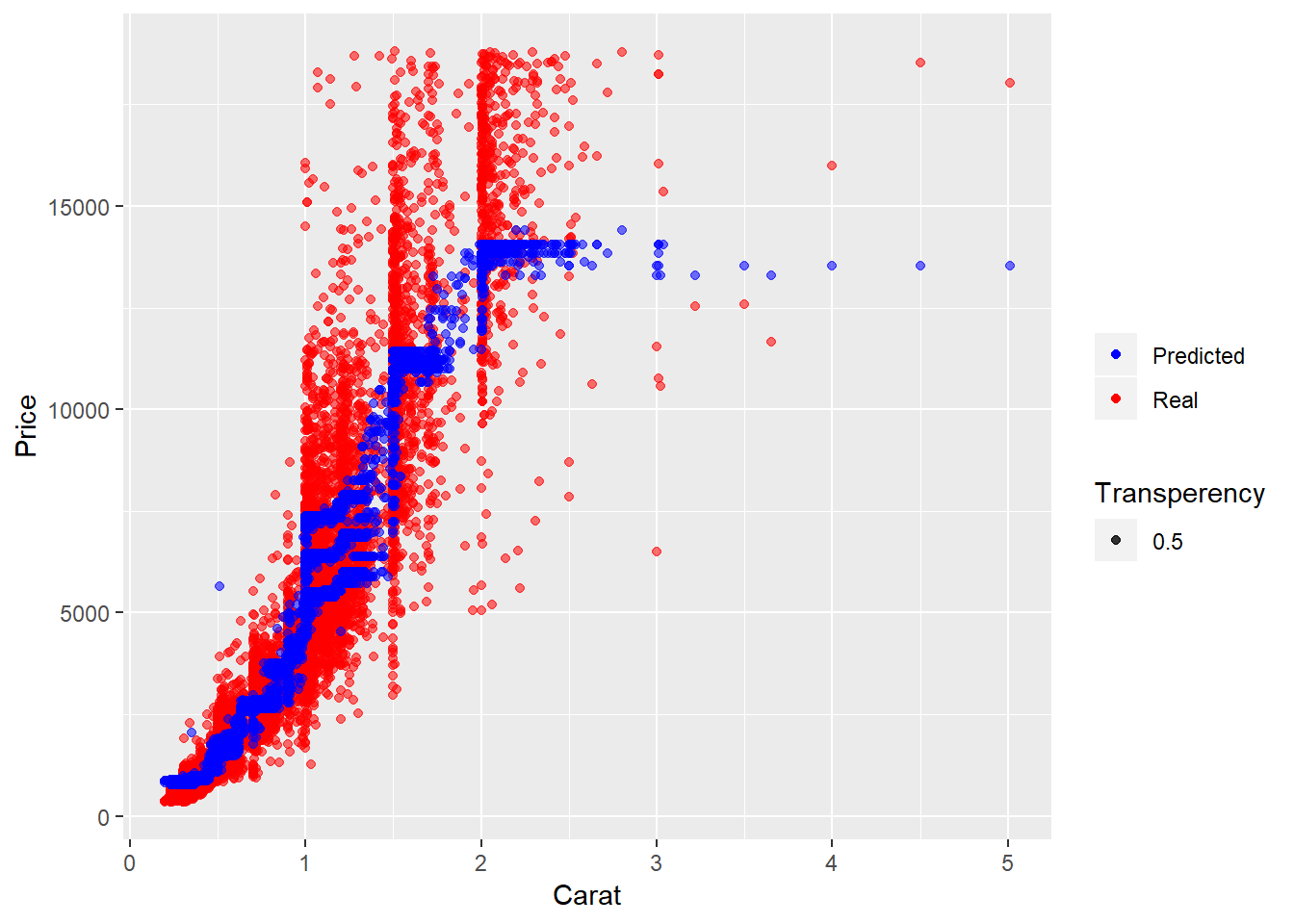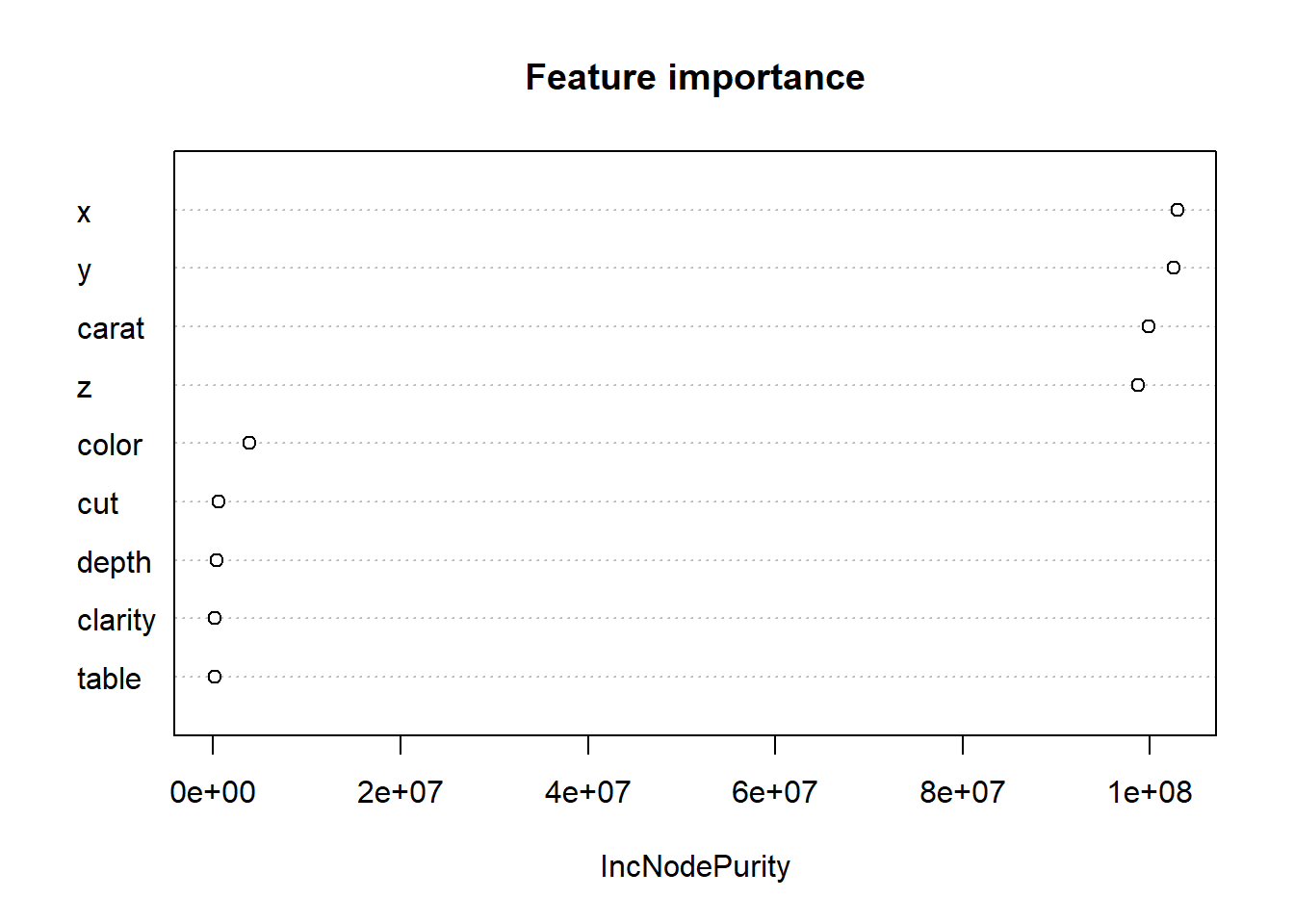[This article was first published on R tutorials – Statistical Aid: A School of Statistics, and kindly contributed to R-bloggers]. (You can report issue about the content on this page here)
Want to share your content on R-bloggers? click here if you have a blog, or here if you don't.

Random Forest is a strong ensemble learning method that may be used to solve a wide range of prediction problems, including classification and regression. Because the method is based on an ensemble of decision trees, it offers all of the benefits of decision trees, such as high accuracy, ease of use, and the absence of the need to scale data. Furthermore, it has a significant advantage over ordinary decision trees in that it is resistant to overfitting as the trees are joined.

In this tutorial, we’ll use a Random Forest Regressor in R to try to forecast the value of diamonds using the Diamonds dataset (part of ggplot2). We examine the tuning of hyperparameters and the relevance of accessible characteristics after visualizing and analyzing the produced prediction model.

```# Import the dataset
diamond <-diamonds
## # A tibble: 6 x 10
##   carat cut       color clarity depth table price     x     y     z
##   <dbl> <ord>     <ord> <ord>   <dbl> <dbl> <int> <dbl> <dbl> <dbl>
## 1 0.23  Ideal     E     SI2      61.5    55   326  3.95  3.98  2.43
## 2 0.21  Premium   E     SI1      59.8    61   326  3.89  3.84  2.31
## 3 0.23  Good      E     VS1      56.9    65   327  4.05  4.07  2.31
## 4 0.290 Premium   I     VS2      62.4    58   334  4.2   4.23  2.63
## 5 0.31  Good      J     SI2      63.3    58   335  4.34  4.35  2.75
## 6 0.24  Very Good J     VVS2     62.8    57   336  3.94  3.96  2.48```

The dataset contains information on 54,000 diamonds. It contains the price as well as 9 other attributes. Some features are in the text format, and we need to encode them in numerical format. Let’s also drop the unnamed index column.

```# Convert the variables to numerical
diamond\$cut <- as.integer(diamond\$cut)
diamond\$color <-as.integer(diamond\$color)
diamond\$clarity <- as.integer(diamond\$clarity)

## # A tibble: 6 x 10
##   carat   cut color clarity depth table price     x     y     z
##   <dbl> <int> <int>   <int> <dbl> <dbl> <int> <dbl> <dbl> <dbl>
## 1 0.23      5     2       2  61.5    55   326  3.95  3.98  2.43
## 2 0.21      4     2       3  59.8    61   326  3.89  3.84  2.31
## 3 0.23      2     2       5  56.9    65   327  4.05  4.07  2.31
## 4 0.290     4     6       4  62.4    58   334  4.2   4.23  2.63
## 5 0.31      2     7       2  63.3    58   335  4.34  4.35  2.75
## 6 0.24      3     7       6  62.8    57   336  3.94  3.96  2.48```

One of the advantages of the Random Forest algorithm is that it does not require data scaling, as previously stated. To apply this technique, all we need to do is define the features and the target we’re attempting to predict. By mixing the available attributes, we might potentially construct a variety of features. We won’t do it right now for the sake of simplicity. If you want to develop the most accurate model, feature creation is a crucial step, and you should devote a significant amount of work to it (e.g. through interaction).

```# Create features and target
X <- diamond %>%
select(carat, depth, table, x, y, z, clarity, cut, color)
y <- diamond\$price```

### Training the model and making predictions

At this point, we have to split our data into training and test sets. As a training set, we will take 75% of all rows and use 25% as test data.

```# Split data into training and test sets
index <- createDataPartition(y, p=0.75, list=FALSE)
X_train <- X[ index, ]
X_test <- X[-index, ]
y_train <- y[index]
y_test<-y[-index]
# Train the model
regr <- randomForest(x = X_train, y = y_train , maxnodes = 10, ntree = 10)```

We now have a model that has been pre-trained and can predict values for the test data. The model’s accuracy is then evaluated by comparing the predicted value to the actual values in the test data. We will present this comparison in the form of a table and plot the price and carat value to make it more illustrative.

```# Make prediction
predictions <- predict(regr, X_test)

result <- X_test
result['price'] <- y_test
result['prediction']<-  predictions

## # A tibble: 6 x 11
##   carat depth table     x     y     z clarity   cut color price prediction
##   <dbl> <dbl> <dbl> <dbl> <dbl> <dbl>   <int> <int> <int> <int>      <dbl>
## 1  0.24  62.8    57  3.94  3.96  2.48       6     3     7   336       881.
## 2  0.23  59.4    61  4     4.05  2.39       5     3     5   338       863.
## 3  0.2   60.2    62  3.79  3.75  2.27       2     4     2   345       863.
## 4  0.32  60.9    58  4.38  4.42  2.68       1     4     2   345       863.
## 5  0.3   62      54  4.31  4.34  2.68       2     5     6   348       762.
## 6  0.3   62.7    59  4.21  4.27  2.66       3     3     7   351       863.
# Import library for visualization
library(ggplot2)

# Build scatterplot
ggplot(  ) +
geom_point( aes(x = X_test\$carat, y = y_test, color = 'red', alpha = 0.5) ) +
geom_point( aes(x = X_test\$carat , y = predictions, color = 'blue',  alpha = 0.5)) +
labs(x = "Carat", y = "Price", color = "", alpha = 'Transperency') +
scale_color_manual(labels = c( "Predicted", "Real"), values = c("blue", "red")) ```The figure displays that predicted prices (blue scatters) coincide well with the real ones (red scatters), especially in the region of small carat values. But to estimate our model more precisely, we will look at Mean absolute error (MAE), Mean squared error (MSE), and R-squared scores.

```# Import library for Metrics
library(Metrics)
##
## Attaching package: 'Metrics'
## The following objects are masked from 'package:caret':
##
##     precision, recall
print(paste0('MAE: ' , mae(y_test,predictions) ))
##  "MAE: 742.401258870433"
print(paste0('MSE: ' ,caret::postResample(predictions , y_test)['RMSE']^2 ))
##  "MSE: 1717272.6547428"
print(paste0('R2: ' ,caret::postResample(predictions , y_test)['Rsquared'] ))
##  "R2: 0.894548902990278"```

We get a couple of errors (MAE and MSE). We should modify the algorithm’s hyperparameters to improve the model’s predictive power. We could do it by hand, but it would take a long time.
We’ll need to build a custom Random Forest model to get the best set of parameters for our model and compare the output for various combinations of the parameters in order to tune the parameters ntrees (number of trees in the forest) and maxnodes (maximum number of terminal nodes trees in the forest can have).

### Tuning the parameters

```# If training the model takes too long try setting up lower value of N
N=500 #length(X_train)
X_train_ = X_train[1:N , ]
y_train_ = y_train[1:N]

seed <-7
metric<-'RMSE'

customRF <- list(type = "Regression", library = "randomForest", loop = NULL)

customRF\$parameters <- data.frame(parameter = c("maxnodes", "ntree"), class = rep("numeric", 2), label = c("maxnodes", "ntree"))

customRF\$grid <- function(x, y, len = NULL, search = "grid") {}

customRF\$fit <- function(x, y, wts, param, lev, last, weights, classProbs, ...) {
randomForest(x, y, maxnodes = param\$maxnodes, ntree=param\$ntree, ...)
}

customRF\$predict <- function(modelFit, newdata, preProc = NULL, submodels = NULL)
predict(modelFit, newdata)
customRF\$prob <- function(modelFit, newdata, preProc = NULL, submodels = NULL)
predict(modelFit, newdata, type = "prob")
customRF\$sort <- function(x) x[order(x[,1]),]
customRF\$levels <- function(x) x\$classes
# Set grid search parameters
control <- trainControl(method="repeatedcv", number=10, repeats=3, search='grid')

# Outline the grid of parameters
tunegrid <- expand.grid(.maxnodes=c(70,80,90,100), .ntree=c(900, 1000, 1100))
set.seed(seed)

# Train the model
rf_gridsearch <- train(x=X_train_, y=y_train_, method=customRF, metric=metric, tuneGrid=tunegrid, trControl=control)
```

### Visualization of Random forest

Let’s visualize the impact of tuned parameters on RMSE. The plot shows how the model’s performance develops with different variations of the parameters. For values maxnodes: 80 and ntree: 900, the model seems to perform best. We would now use these parameters in the final model.

`plot(rf_gridsearch)`#### Best parameters

```rf_gridsearch\$bestTune
##   maxnodes ntree
## 5       80  1000```

#### Defining and visualizing variables importance

For this algorithm, we used all available diamond features, but some of them contain more predictive power than others.

Let’s build the plot with a features list on the y axis. On the X-axis we’ll have an incremental decrease in node impurities from splitting on the variable, averaged over all trees, it is measured by the residual sum of squares and therefore gives us a rough idea about the predictive power of the feature. Generally, it is important to keep in mind, that random forest does not allow for any causal interpretation.

`varImpPlot(rf_gridsearch\$finalModel, main ='Feature importance')`From the figure above you can see that the size of the diamond (x,y,z refer to length, width, depth) and the weight (carat) contains the major part of the predictive power.

Learn Data Science and Machine learning

The post Random Forest in R appeared first on Statistical Aid: A School of Statistics.